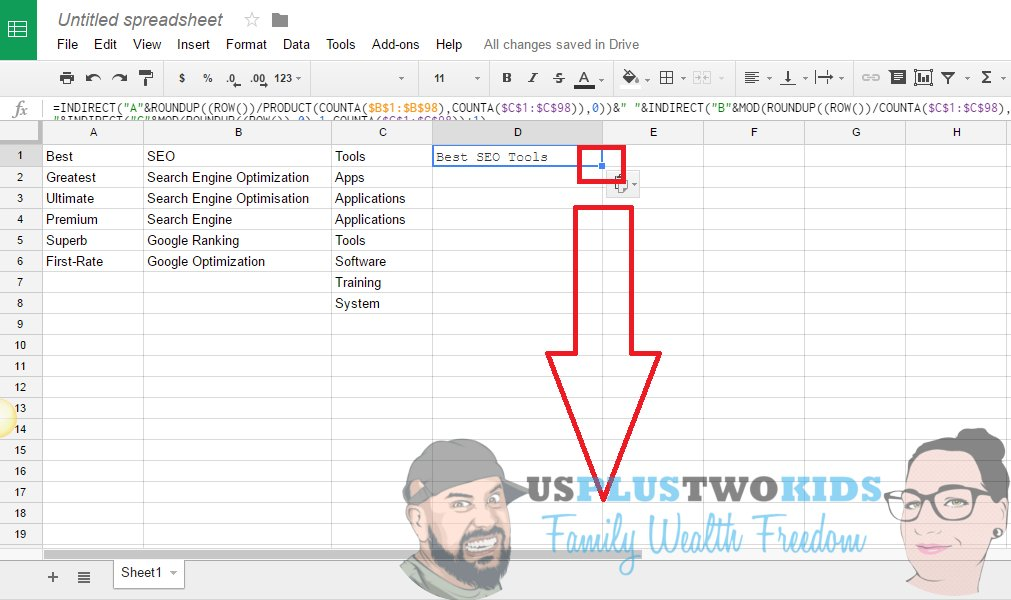Next up we need to add this formula to a blank space in the spreadsheet

=INDIRECT("A"&ROUNDUP((ROW())/PRODUCT(COUNTA(\$B\$1:\$B\$98),COUNTA(\$C\$1:\$C\$98)),0))&" "&INDIRECT("B"&MOD(ROUNDUP((ROW())/COUNTA(\$C\$1:\$C\$98),0)-1,COUNTA(\$B\$1:\$B\$98))+1)&" "&INDIRECT("C"&MOD(ROUNDUP((ROW()),0)-1,COUNTA(\$C\$1:\$C\$98))+1)In the above image I have pasted the code in the cell that is highlighted. As you can see it has produced the term "Best SEO Tools".

The next stage is to drag the formula down.Keep dragging this down until all the different keyword variations have been produced. A quick maths equation will tell you how many different variations you will produce.

Total the amount of words in each column. In this case we have:

• A = 6
• B = 6
• C = 8

This makes the equation: 6 x 6 x 8 = 288 keyword variations!!!

Join the Discussion
Recent messages